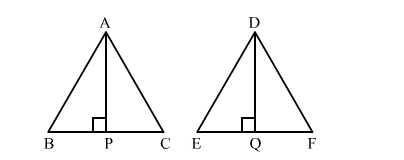# ∆ABC ∼ ∆DEF and their areas are respectively 100 cm2 and 49 cm2.

Question:

ABC ∼ ∆DEF and their areas are respectively 100 cm2 and 49 cm2. If the altitude of ∆ABC is 5 cm, find the corresponding altitude of ∆DEF.

Solution:It is given that ∆ABC ∼ ∆DEF.
Therefore, the ratio of the areas of these triangles will be equal to the ratio of squares of their corresponding sides.
Also, the ratio of areas of two similar triangles is equal to the ratio of squares of their corresponding altitudes.

Let the altitude of
ABC be AP, drawn from A to BC to meet BC at P and the altitude of ∆DEF be DQ, drawn from D to meet EF at Q.

Then,

$\frac{\operatorname{ar}(\Delta A B C)}{\operatorname{ar}(\Delta D E F)}=\frac{A P^{2}}{D Q^{2}}$

$\Rightarrow \frac{100}{49}=\frac{5^{2}}{D Q^{2}}$

$\Rightarrow \frac{100}{49}=\frac{25}{D Q^{2}}$

$\Rightarrow D Q^{2}=\frac{49 \times 25}{100}$

$\Rightarrow D Q=\sqrt{\frac{49 \times 25}{100}}$

$\Rightarrow D Q=3.5 \mathrm{~cm}$

Hence, the altitude of DEF is 3.5 cm